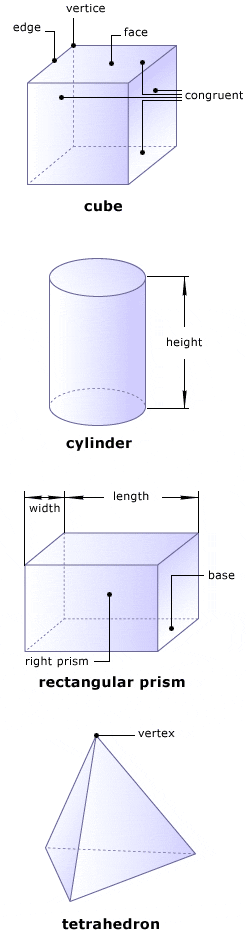# Glossaryapex
in a pyramid, the vertex at which all lateral faces meetbase
in a prism, the two congruent, parallel faces at the top and bottom; in a pyramid, the regular polygon that is not a lateral facecongruent
having the same size and shapecube
a polyhedron with six congruent, square facescylinder
a three-dimensional object with circular basesdodecahedron
a polyhedron with 12 congruent, pentagonal facesedge
the segment along which two faces meetEuler's Theorem
for any polyhedron, the sum of the number of vertices and faces is equal to two more than the number of edges; stated another way, F + V - E = 2face
one of the surfaces of a polyhedronheight
the distance from top to bottom of a three-dimensional figureicosahedron
a polyhedron with 20 congruent, triangular faceslateral face
in a prism, one of the rectangles that connects the bases to one another; in a pyramid, one of the triangles that connects the base to the apexlength
the longest dimension of a solid figure; for a three-dimensional figure, it is usually the distance from front to backoctahedron
a polyhedron with eight congruent, triangular facespolygon
a two-dimensional figure formed by connecting three or more straight line segments end to end (triangles, squares, and hexagons are examples of polygons)polyhedron
a three-dimensional figure whose faces are polygonsprism
a three-dimensional figure with two parallel, congruent bases connected by rectanglesrectangular prism
a prism whose bases are rectangles; in general, a prism can be described by the shape of its basesright prism
a prism in which the bases meet the lateral faces at right anglestetrahedron
a polyhedron with four congruent, triangular facestruncated icosahedron
a polyhedron with 32 faces, 12 of which are pentagons and 20 of which are hexagonsvertex vertices[plural]
the point at which three or more faces meetwidth
distance from side to side